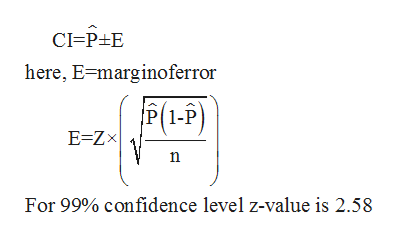# Out of 300 people sampled, 78 preferred Candidate A. Based on this, estimate what proportion of the entire voting population (p) prefers Candidate A.Use a 99% confidence level, and give your answers as decimals, to three places. ____< pp < ______

Question
8 views

Out of 300 people sampled, 78 preferred Candidate A. Based on this, estimate what proportion of the entire voting population (p) prefers Candidate A.

Use a 99% confidence level, and give your answers as decimals, to three places.

____< pp < ______

check_circle

Step 1

From the given information,

Out of 300 people sampled, 78 preferred candidate A.

So, here n= 300, x=78

Step 2

Formula for confidence interval for population proportion is,help_outlineImage TranscriptioncloseCI P E here, E marginoferror P 1-P) E Zx For 99% confidence level z-value is 2.58 fullscreen
Step 3

&n...

### Want to see the full answer?

See Solution

#### Want to see this answer and more?

Solutions are written by subject experts who are available 24/7. Questions are typically answered within 1 hour.*

See Solution
*Response times may vary by subject and question.
Tagged in

### Statistics